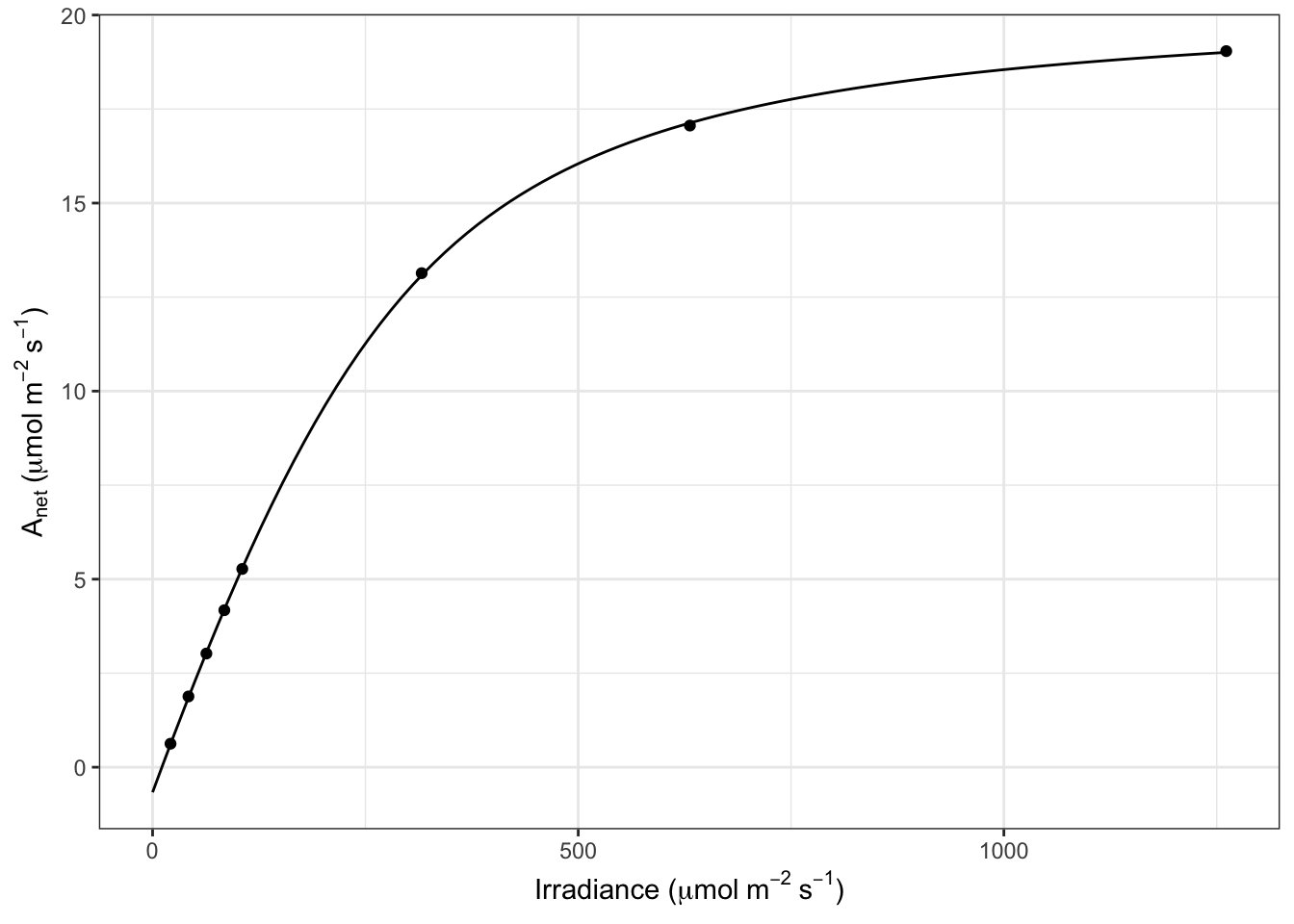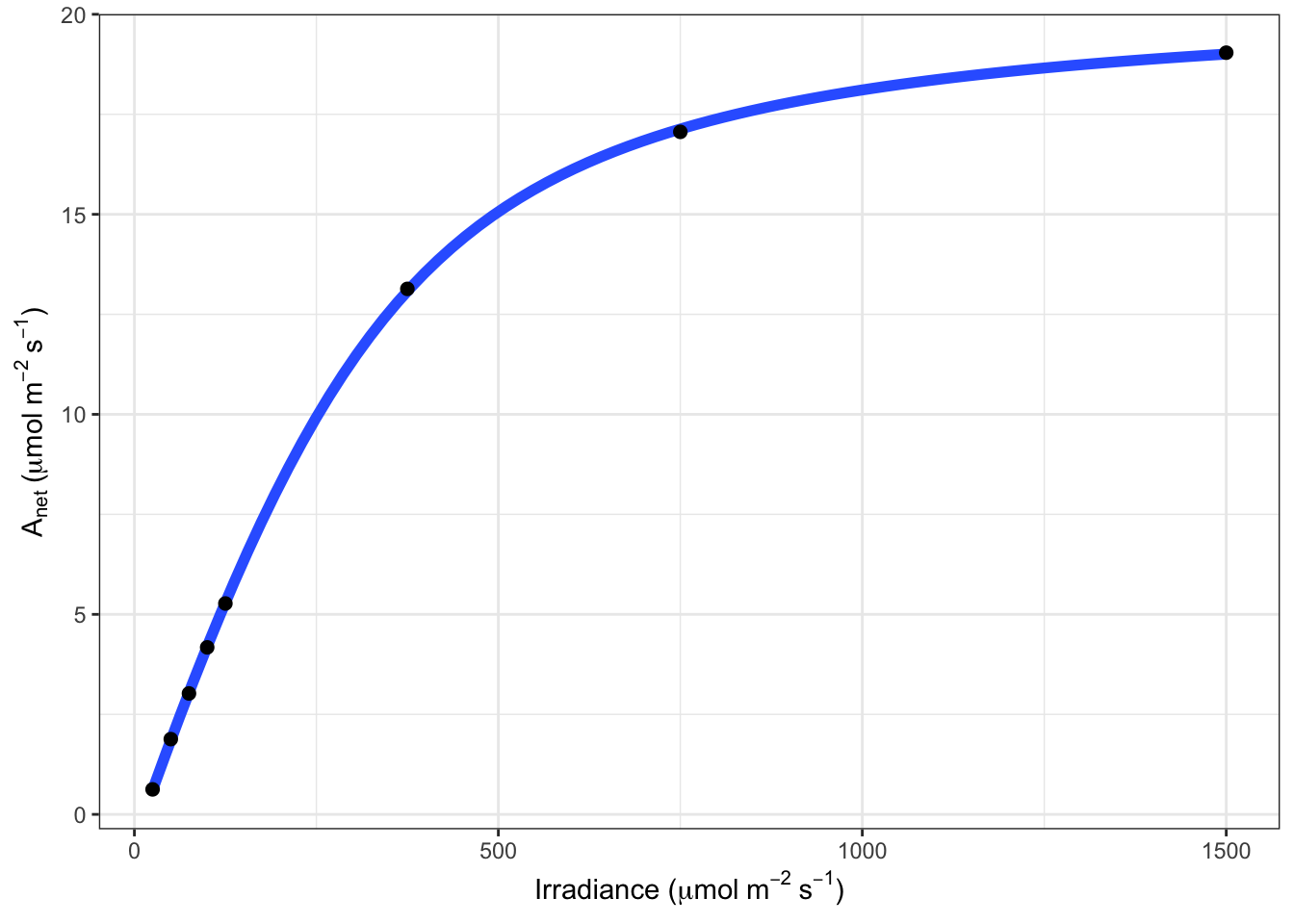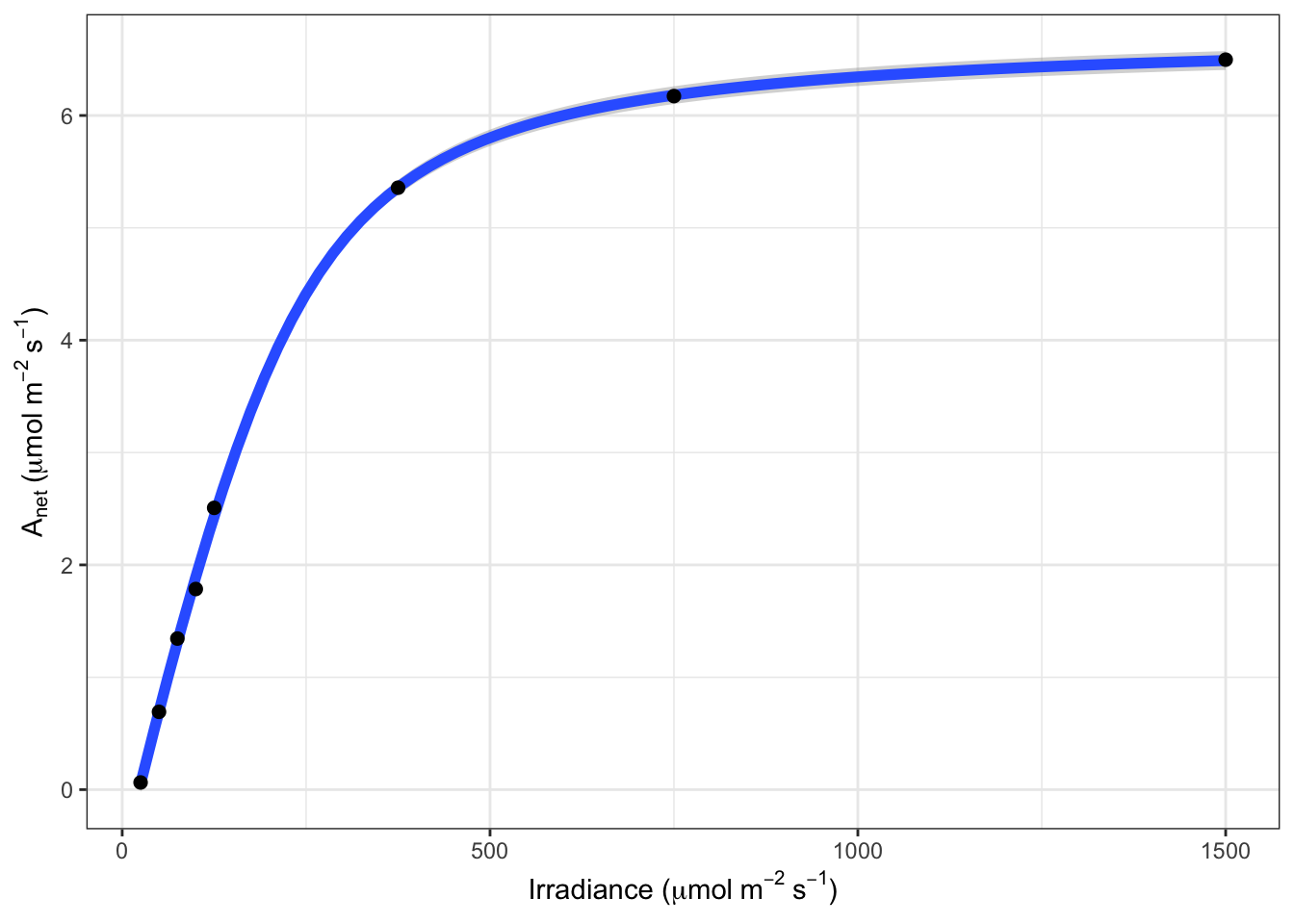## Preferred version (photosynthesis >= 2.1.1)

This package currently only implements the Marshall & Biscoe (1980) non-rectangular hyperbola model of the photosynthetic light response.

### Fit the light-response curve using nonlinear least-squares (nls)

library(broom)
library(dplyr)
library(photosynthesis)

dat = system.file("extdata", "A_Ci_Q_data_1.csv", package = "photosynthesis") |>
# Set grouping variable
mutate(group = round(CO2_s, digits = 0)) |>
# For this example, round sequentially due to CO2_s set points
mutate(group = as.factor(round(group, digits = -1)))

# Fit one light-response curve
fit = fit_photosynthesis(
.data = filter(dat, group == 600),
.photo_fun = "aq_response",
.vars = list(.A = A, .Q = Qabs),
)

# The 'fit' object inherits class 'nls' and many methods can be used

## Model summary:
summary(fit)
##
## Formula: .A ~ marshall_biscoe_1980(Q_abs = .data[[".Qabs"]], k_sat, phi_J,
##     theta_J) - Rd
##
## Parameters:
##          Estimate Std. Error t value Pr(>|t|)
## k_sat   21.170337   0.154428  137.09 1.70e-08 ***
## phi_J    0.061543   0.001218   50.52 9.19e-07 ***
## theta_J  0.775752   0.014526   53.40 7.36e-07 ***
## Rd       0.666320   0.063490   10.49 0.000466 ***
## ---
## Signif. codes:  0 '***' 0.001 '**' 0.01 '*' 0.05 '.' 0.1 ' ' 1
##
## Residual standard error: 0.0539 on 4 degrees of freedom
##
## Number of iterations to convergence: 5
## Achieved convergence tolerance: 1.49e-08
## Estimated parameters:
coef(fit)
##       k_sat       phi_J     theta_J          Rd
## 21.17033721  0.06154348  0.77575157  0.66631987
## 95% confidence intervals:
confint(fit)
##                2.5%       97.5%
## k_sat   20.75337772 21.60612987
## phi_J    0.05835624  0.06501655
## theta_J  0.73285635  0.81306156
## Rd       0.49523165  0.84311606
## Tidy summary table using 'broom::tidy()'
tidy(fit, conf.int = TRUE, conf.level = 0.95)
## # A tibble: 4 × 7
##   term    estimate std.error statistic      p.value conf.low conf.high
##   <chr>      <dbl>     <dbl>     <dbl>        <dbl>    <dbl>     <dbl>
## 1 k_sat    21.2      0.154       137.  0.0000000170  20.8      21.6
## 2 phi_J     0.0615   0.00122      50.5 0.000000919    0.0584    0.0650
## 3 theta_J   0.776    0.0145       53.4 0.000000736    0.733     0.813
## 4 Rd        0.666    0.0635       10.5 0.000466       0.495     0.843
## Calculate light compensation point
coef(fit) |>
t() |>
as.data.frame() |>
mutate(LCP = ((Rd) * (Rd * theta_J - k_sat) / (phi_J * (Rd - k_sat)))) |>

## Calculate residual sum-of-squares
sum(resid(fit) ^ 2)
##  33.59129

## Plot model fit and raw data

The deprecated function fit_aq_response() generated a figure automatically, but it used geom_smooth() rather than plotting the model fit. We now prefer to use generic methods from the package ggplot2 and plot the fitted curve. This allows users the ability to more easily customize their figures.

library(ggplot2)

b = coef(fit)

df_predict = data.frame(Qabs = seq(0, 0.84 * 1500, length.out = 100)) |>
mutate(
A = marshall_biscoe_1980(
Q_abs = Qabs,
k_sat = b["k_sat"],
b["phi_J"],
b["theta_J"]
) - b["Rd"]
)

ggplot(mapping = aes(Qabs, A)) +
geom_line(data = df_predict) +
geom_point(data = filter(dat, group == 600)) +
labs(
x = expression("Irradiance (" * mu * mol ~ m^{-2} ~ s^{-1} * ")"),
y = expression(A[net] ~ "(" * mu * mol ~ m^{-2} ~ s^{-1} * ")")
) +
theme_bw()## Fit multiple curves with photosynthesis and purrr

In the previous version, we used fit_many() to fit many light-response curves simultaneously. We now prefer to use generic methods from the package purrr that are already pretty good.

library(purrr)

fits = dat |>
split(~ group) |>
map(fit_photosynthesis, .photo_fun = "aq_response", .vars = list(.A = A, .Q = Qabs))

## Estimated parameters:
fits |>
map(coef) |>
map(t) |>
map(as.data.frame) |>
imap_dfr(~ mutate(.x, CO2_s = .y))
##        k_sat       phi_J   theta_J          Rd CO2_s
## 1 -0.1832198 0.004189779 0.2428476 -0.09209662    50
## 2  2.6370733 0.017295931 0.8858139  0.59492313   100
## 3  7.3481571 0.032255707 0.8376462  0.61479141   200
## 4 11.4687982 0.045046336 0.7898826  0.76746932   300
## 5 16.4505827 0.057537264 0.6997933  0.89736502   410
## 6 21.1703372 0.061543475 0.7757516  0.66631987   600
## 7 23.9305140 0.067744069 0.7514331  0.78493847   820
## 8 25.0686239 0.068975627 0.7632357  0.58529680  1200
## 9 25.6413999 0.071556328 0.7411443  0.45326420  1600

## Fit Bayesian light-response curves with brms and Stan

Traditional model fitting use a nonlinear least-squares approach, but Bayesian methods have some advantages, especially with more complex data sets. We added an option to fit a single Bayesian light-response curve using the amazing brms package which fits models in Stan. We have not implemented more complex approaches (e.g. multilevel light-response models) because once you are doing that, it’s probably easier to code the model directly into brms functions. Hopefully this code can get you started though. We have not run the example below, but copy-and-paste into your R Console to try.

fit = fit_photosynthesis(
.data = filter(dat, group == 600),
.photo_fun = "aq_response",
.vars = list(.A = A, .Q = Qabs),
.method = "brms",
brm_options = list(chains = 1)
)

# The 'fit' object inherits class 'brmsfit' and many methods can be used
summary(fit)

## Deprecated version (photosynthesis <= 2.1.1)

The fit_aq_response() function is the original version, but we are no longer updating it and may phase it out of future releases. Use fit_photosynthesisi(..., .photo_fun = 'aq_response') instead.

library(dplyr)
library(photosynthesis)
dat = system.file("extdata", "A_Ci_Q_data_1.csv", package = "photosynthesis") |>
# Set grouping variable
mutate(group = round(CO2_s, digits = 0)) |>
# For this example, round sequentially due to CO2_s setpoints
mutate(group = as.factor(round(group, digits = -1))) |>
rename(A_net = A, PPFD = Qin)

# To fit one AQ curve
fit = fit_aq_response(filter(dat, group == 600))
## Warning: fit_aq_response() was deprecated in photosynthesis 2.1.1.
## ℹ Please use the .photo_fun argument of fit_photosynthesis() instead.
## This warning is displayed once every 8 hours.
## Call lifecycle::last_lifecycle_warnings() to see where this warning was
## generated.
## Warning: aq_response() was deprecated in photosynthesis 2.1.1.
## ℹ Please use marshall_biscoe_1980() instead.
## ℹ The deprecated feature was likely used in the photosynthesis package.
##   Please report the issue at <https://github.com/cdmuir/photosynthesis/issues>.
## This warning is displayed once every 8 hours.
## Call lifecycle::last_lifecycle_warnings() to see where this warning was
## generated.
# Print model summary
summary(fit[])
##
## Formula: A_net ~ aq_response(k_sat, phi_J, Q_abs = data$Q_abs, theta_J) - ## Rd ## ## Parameters: ## Estimate Std. Error t value Pr(>|t|) ## k_sat 21.167200 0.158332 133.69 1.88e-08 *** ## phi_J.Q_abs 0.051907 0.001055 49.18 1.02e-06 *** ## theta_J 0.775484 0.014920 51.98 8.20e-07 *** ## Rd.(Intercept) 0.668495 0.065235 10.25 0.000511 *** ## --- ## Signif. codes: 0 '***' 0.001 '**' 0.01 '*' 0.05 '.' 0.1 ' ' 1 ## ## Residual standard error: 0.05535 on 4 degrees of freedom ## ## Number of iterations to convergence: 5 ## Achieved convergence tolerance: 1.49e-08 # Print fitted parameters fit[] ## A_sat phi_J theta_J Rd LCP resid_SSs ## k_sat 21.1672 0.05190746 0.7754836 0.6684953 12.97289 0.01225491 # Print graph fit[]# Fit many curves fits = fit_many( data = dat, funct = fit_aq_response, group = "group", progress = FALSE ) # Look at model summary for a given fit # First set of double parentheses selects an individual group value # Second set selects an element of the sublist summary(fits[][]) ## ## Formula: A_net ~ aq_response(k_sat, phi_J, Q_abs = data$Q_abs, theta_J) -
##     Rd
##
## Parameters:
##                Estimate Std. Error t value Pr(>|t|)
## k_sat          7.347423   0.141931  51.768 8.33e-07 ***
## phi_J.Q_abs    0.027192   0.001511  17.994 5.61e-05 ***
## theta_J        0.837778   0.030608  27.371 1.06e-05 ***
## Rd.(Intercept) 0.615283   0.086994   7.073  0.00211 **
## ---
## Signif. codes:  0 '***' 0.001 '**' 0.01 '*' 0.05 '.' 0.1 ' ' 1
##
## Residual standard error: 0.06799 on 4 degrees of freedom
##
## Number of iterations to convergence: 4
## Achieved convergence tolerance: 1.49e-08
# Print the parameters
fits[][]
##          A_sat      phi_J   theta_J        Rd      LCP  resid_SSs
## k_sat 2.637157 0.01458002 0.8858892 0.5951635 42.17813 0.02446394
# Print the graph
fits[][]#Compile graphs into a list for plotting
fits_graphs = compile_data(fits, list_element = 3)

# Print graphs to jpeg
# print_graphs(data = fits_graphs, path = tempdir(), output_type = "jpeg")

#Compile parameters into data.frame for analysis
fits_pars = compile_data(fits, output_type = "dataframe", list_element = 2)